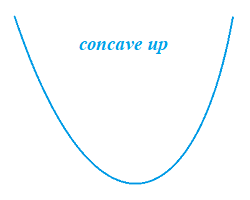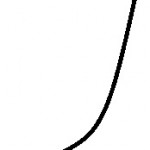# Concave Up (Convex), Down (Function)

Contents:

1. What Do “Concave Up” and “Concave Down” Mean?
2. What is a Concave Function?
1. ## What Do “Concave Up” and “Concave Down” Mean?

Concave up (also called convex) or concave down are descriptions for a graph, or part of a graph:

• A concave up graph looks roughly like the letter U
• A concave down graph is shaped like an upside down U (“⋒”).

They tell us something about the shape of a graph, or more specifically, how it bends. That kind of information is useful when it comes to analyzing graphs using derivatives.

A concave up graph has the “U” or bowl right-side up.Note though, that it doesn’t have to look like a perfect “U”: it can be a flattened, scrunched, or stretched. It might even be more like a half of a U, like this:On the other hand, a concave down graph has the letter U or bowl upside down, so that it looks more like a cap (∩).As with concave up, the upside down “U” in a concave down graph can also be flattened, scrunched, or stretched.

## Defining Concave Up or Down with Tangent Lines

Analytically, a concave up graph can be defined by its tangent line; Take a point where the graph has a low point: the tangent line around that point lies below the graph.On the other hand, if the tangent line is above the point, then the graph is concave down.The slopes of tangent lines can also tell you which type of concavity you have. In general, the slopes of the tangent lines increase (from left to right) when the graph’s concavity is up.If the slopes of the tangent lines decrease (from left to right) then you most likely have a concave down graph.

## Using Derivative Tests to Show Concavity

The first derivative test and second derivative test can be used to determine a graph’s concavity, as well as if the function is decreasing or increasing at that point. The idea is that you find the first derivative, then find the second derivative. The signs of the results tell you whether your function is concave up or concave down (as well as whether it’s an increasing or decreasing function . The following table summarizes the information given by the two tests:## What is a Concave Function?

A concave function has a distinct arched appearance when graphed:The term concave down is sometimes used as a synonym for concave function. However, the usual distinction between the two is that “concave down” refers to the shape of a graph, or part of a graph. While some functions can have parts that are concave up and other parts that are concave down, a concave function is concave up for its entire domain.

Concave functions are very useful in maximization problems (like profit maximization), because all you have to do to find the solution is to find where the “peak” of the function is.

## Definition of a Concave Function

A concave function can be defined directly in terms of convex functions. They can also be defined graphically.

1. In Terms of Convex Functions: Gradshteyn and Ryzhik (2000) state the relationship with convex functions more mathematically: A function is concave on some interval [a,b] if, for any points x1 and x2 in that interval, the function -f(x) is convex.
2. Graphical Definition: A concave function can also be defined graphically, in comparison to a convex function. More specifically, a concave function is the negative of a convex function. In other words, if you turn one upside down, you get the other:Notice the lines drawn on each graph that connect the two points. Graphically, a concave function is defined as one where no line segment joining any two points on the graph lies above the curve of the graph. Convex functions are defined similarly, except the line segments all lie above the curve.

## References

Gradshteyn, I. S. and Ryzhik, I. M. Tables of Integrals, Series, and Products, 6th ed. San Diego, CA: Academic Press, p. 1132, 2000.

CITE THIS AS:
Stephanie Glen. "Concave Up (Convex), Down (Function)" From StatisticsHowTo.com: Elementary Statistics for the rest of us! https://www.statisticshowto.com/concave-up-down/